nextnano.com  nextnano³  Download | Search | Copyright | Publications  * password protected nextnano³ softwareOptical absorption

www.nextnano.com/documentation/tools/nextnano3/reference/input_file/keywords/optical-absorption.html

# Optical absorption

Detailed description about the Physics: Absorption, Matrix elements, Inter-band transitions, Intra-band transitions.

A tutorial is available that describes this keyword: Optical absorption of an InGaAs quantum well

Here you can specify the options for the optical absorption.
This will be a 3-step approach:

• Step 1:``` calculate-optics = no```
Solve k.p and determine lowest and highest eigenvalue to specify``` e-min-state ```and` e-max-state` in the second step.
• Step 2:``` calculate-optics = yes read-in-k-points = no ```Read in raw data (potential, quasi-Fermi levels, strain (if applicable)) and output k points:
```raw-potential-in = yes, raw-fermi-levels-in = yes, strain-calculation = raw-strain-in```
• Step 3: Calculate optical absorption``` calculate-optics = yes read-in-k-points = yes ```Read in k points, calculate and output optical absorption.
If one wants to repeat the calculation for another polarization, one only needs to change the polarization vector and repeat step 3.
It is not necessary in this case to recalculate step 1 or step 2.
Step 3 also outputs the energy dispersion E(k||) = E(kx,ky).

```!---------------------------------------------------------! \$optical-absorption                             optional  !  destination-directory            character     required  !  calculate-optics                 character     optional  ! ```flag:``` "yes"/"no"  kind-of-absorption               character     optional  !  read-in-k-points                 character     optional  ! ```flag:``` "yes"/"no"                                                           !  ! ```if` 'read-in-k-points = no' `then specify k point calculation`       ! ` 2nd step```  num-quantum-cluster              integer       optional  ! ```(optional)```  e-min-state                      double        optional  ! [eV] ```lowest  eigenvalue```  e-max-state                      double        optional  ! [eV] ```highest eigenvalue```                                                           !                                                           ! ```3rd step```  e-min-photon                     double        optional  ! [eV] ```lower  boundary```  e-max-photon                     double        optional  ! [eV] ```uppper boundary```  num-energy-steps                 integer       optional  !  smoothing-of-curve               character     optional  ! ```flag:``` "yes"/"no"  smoothing-damping-parameter      double        optional  ! [eV] ```artificial damping parameter for smoothing of absorption curve```  E-P                              double        optional  ! [eV]  |<S|p|X>|^2  polarization-vector-1            double_array  optional  ! x y z ```coordinates```  polarization-vector-2            double_array  optional  ! x y z ```coordinates```  magnitude-relation-1-2           double        optional  ! |E1|/|E2|  phase                            double        optional  ! E2 -> exp(i*phase*Pi)*E2                                                           !  fermi_in_el                      double        optional  ! [eV] ```optional input for Fermi level of electrons (default: calculated Fermi level)```  fermi_in_hl                      double        optional  ! [eV] ```optional input for Fermi level of holes      (default: calculated Fermi level)```  device_thickness_in              double        optional  ! [m]  ```optional input of device thickness for normalization of absorption```                                                           !  k-space-symmetry                 character     optional  !                                                           !                                                           !  incident-light-along-direction   character     optional  ! x,y,z                                                           !  import-absorption-spectrum       character     optional  ! ```flag:``` "yes"/"no"  file-absorption-spectrum         character     optional  !                                                          !  import-reflectivity-spectrum     character     optional  ! ```flag:``` "yes"/"no"  file-reflectivity-spectrum       character     optional  !                                                          !  import-transmission-spectrum     character     optional  ! ```flag:``` "yes"/"no"  file-transmission-spectrum       character     optional  !                                                          !  import-solar-spectrum            character     optional  ! ```flag:``` "yes"/"no"  file-solar-spectrum              character     optional  !                                                           !  number-of-suns                   double        optional  !                                                           !  calculate-black-body-spectrum    character     optional  ! ```flag:``` "yes"/"no" \$end_optical-absorption                         optional  ! !---------------------------------------------------------!```

## Syntax

```destination-directory  = optics1/ ```Directory for output and data files.

```calculate-optics       = yes                        = no ```Flag to distinguish between
- Step 1: For calculation of k.p wavefunctions for all k|| vectors), and
- Step 2 and Step 3: Reading in previously calculated k.p wavefunctions for all k|| vectors (in order to save CPU time), and then calculating the optical absorption.

```e-min-photon           = 1.500      ! [eV] ```lower boundary for photon energy``` e-max-photon           = 1.700      ! [eV] ```upper boundary for photon energy``` ```Specifications for absorption energy range: lower/upper boundary for photon energy interval

```num-energy-steps       = 1000 ```Number of energy steps between` e-min-photon `and``` e-max-photon```.
This number determines the resolution of the absorption curve alpha(E) where E is the energy in units of [eV].
`==> `The number of energy grid points = ```num-energy-steps + 1 ```because the first grid point (`e-min-photon`) is also included.

First step

```kind-of-absorption     = interband-only                        = intra-vb-only                        = intra-cb-only                        = intra-sg-only 'interband-only': ```considers only interband transitions between holes and electrons```                        heavy hole     <-->   Gamma band                        light hole     <-->   Gamma band                        split-off hole <-->   Gamma band 'intra-vb-only' : ```considers only intraband transitions within the valence bands```                        heavy hole     <-->   light hole                        heavy hole     <-->   split-off hole                        light hole     <-->   split-off hole                        heavy hole     <-->   heavy hole                        light hole     <-->   light hole                        split-off hole <-->   split-off hole 'intra-cb-only' : ```considers only intraband transitions within the conduction band (Gamma band)```                        Gamma band     <-->   Gamma band 'intra-sg-only' : ```considers only intraband transitions within the same band (single-band for Gamma, L, X, heavy hole, light hole, split-off hole band)```                        Gamma band     <-->   Gamma band                        L band         <-->   L band                        X band         <-->   X band                        heavy hole     <-->   heavy hole                        light hole     <-->   light hole                        split-off hole <-->   split-off hole                    ``` This is a simple algorithm taking only account the energy levels and wave functions at k||=0.

```read-in-k-points       = yes                        = no ```Flag to distinguish between step 2 and 3.

Second step

If` 'read-in-k-points = no' `then specify k point calculation. (These parameters will be ignored in Step 3.)

```num-quantum-cluster = 1 ! ```(optional)``` ```Number of quantum cluster for which absorption is calculated.
If this specifier is not present, the default value (`num-quantum-cluster = 1`) is taken.

```e-min-state            = -1.7      ! [eV] ```lowest  eigenvalue``` e-max-state            =  0.3      ! [eV] ```highest eigenvalue``` ```energy of lowest/highest eigenvalue considered for calculation

`\$quantum-model-electrons`
``` ...  num-kp-parallel  = 1700   ! STEP 2/3   ! ```total number of k|| points for Brillouin zone discretization``` \$quantum-model-holes```
``` ...  num-kp-parallel  = 1700   ! STEP 2/3   ! ```total number of k|| points for Brillouin zone discretization``` ```The user specifies the total number of k|| points that are present in k|| space.
However, internally the code modifies this number according to the following algorithm:
`- `number of k points in positive x direction (without Gamma point): N_kx
`- `number of k points in positive y direction (without Gamma point): N_ky = N_kx
`==> `Thus the actual, total number of k|| points is:
total_number_of_k|| = (2 * N_kx + 1) * (2 * N_ky + 1)
In this example (`num-kp-parallel = 1700`):
`- `N_kx = N_ky = 20
`==> `total_number_of_k|| = 41 * 41 = 1681

Third step

`'read-in-k-points = yes' `to read in k points, calculate and output optical absorption.

```smoothing-of-curve          = yes     ! ```(default)```                             = no      ! smoothing-damping-parameter = 0.5e-3  ! [eV] ```(only relevant for the calculation of the absorption curve in the 3rd step)``` ```Smoothing of absorption curve: We introduce an artificial smoothing of the curve by calling the function` L``orentzian`.
The artificial parameter for smoothing of absorption curve is``` smoothing-damping-parameter```. It is usually denoted as Gamma and is the Full Width at Half Maximum (FWHM).

```  absorption_NoSmoothingV = absorptionV   absorptionV = 0.0   DO  i=1,num-energy-steps+1 ! Loop over all energy grid points E(i) and determine absorption alpha(i)=alpha(E).    DO j=1,num-energy-steps+1 ! This loop is essentially an integration over energy dE.     E_weight = (Lorentzian( E_gridV(j),   E_gridV(i), smoothing-damping-parameter ) - &                 Lorentzian( E_gridV(j), - E_gridV(i), smoothing-damping-parameter ) ) * DeltaEnergy     absorptionV(i) = absorptionV(i) + absorption_NoSmoothingV(j) * E_weight    END DO   END DO ```
Lorentzian lineshape

```   FUNCTION Lorentzian (E,E0,Gamma)             Lorentzian = 1 / pi * alpha / ( (E - E0)2 + alpha2 )             alpha ``` is the scale parameter 'Lorentzian half-width': Half Width at Half Maximum (HWHM)```             alpha = Gamma / 2 = smoothing-damping-parameter / 2    DeltaEnergy = (e-max-photon - e-min-photon ) / (num-energy-steps)    E_gridV = ```energy of light for which absorption should be calculated``` [eV]      DO i=1,num-energy-steps+1       E_gridV(i) = e-min-photon + (i-1) * DeltaEnergy      END DO  ```

The following specifiers are only used for the k.p optical absorption algorithm but not for the simple intersubband transition algorithm.

```E_P                    = 22.0        ! [eV] E_P ```is Kane's matrix element EP = | < S | p | X > |2. It should be around 20 eV and depends on the material.
The` E_P [eV] `parameter is given in the database by the specifier``` 8x8kp-parameters```.
` E_P `can be converted into the` P `paramter by the following equation:
```EP = 2m0/hbar2 * P2 ```In our model the` E_P `parameter is only relevant for interband transitions. It enters into the matrix element prefector (`matrix_element_prefac`) which is described in Section "1.1.1 Inter-band transitions" of the documentation:
Absorption, Matrix elements, Inter-band transitions, Intra-band transitions.
`E_P `has the same value for all materials in this implementation. In principle it could have been read in from the database rather than specifying it within the keyword
``` \$optical-absorption```.

```polarization-vector-1  = 1.0  0.0  0.0 polarization-vector-2  = 0.0  1.0  0.0 x y z ```coordinates (in simulation system) for first/second in-plane vector

Example:

•  1D well, inter-band
```polarization-vector-1  = 1.0  0.0  0.0 polarization-vector-2  = 0.0  1.0  0.0 magnitude-relation-1-2 = 1.0```
•  1D well, intra-band
```polarization-vector-1  = 0.0  0.0  1.0 polarization-vector-2  = 0.0  0.0  1.0 magnitude-relation-1-2 = 0.0 ```Note: Intraband absorption only for z-polarized light.

How to specify x-polarized light:
``` polarization-vector-1  = 0.0 1.0 0.0    ! ```x y z coordinates (in simulation system) for first in-plane vector```  polarization-vector-2  = 1.0 0.0 0.0    ! ```x y z coordinates (in simulation system) for second in-plane vector```  magnitude-relation-1-2 = 0.0            ! ```relation of magnitudes``` |E1|/|E2|  ```(In this case,` polarization-vector-1 `is ignored as``` |E1| ```is set to be zero.)``` ```How to specify z-polarized light:
``` polarization-vector-1  = 1.0 0.0 0.0    ! ```x y z coordinates (in simulation system) for first in-plane vector```  polarization-vector-2  = 0.0 0.0 1.0    ! ```x y z coordinates (in simulation system) for second in-plane vector```  magnitude-relation-1-2 = 0.0            ! ```relation of magnitudes``` |E1|/|E2|  ```(In this case,` polarization-vector-1 `is ignored as``` |E1| ```is set to be zero.)``` ```How to specify circularly polarized light [(x,z) plane]:
``` polarization-vector-1  = 1.0 0.0 0.0    ! ```x y z coordinates (in simulation system) for first in-plane vector```  polarization-vector-2  = 0.0 0.0 1.0    ! ```x y z coordinates (in simulation system) for second in-plane vector```  magnitude-relation-1-2 = 1.0            ! ```relation of magnitudes``` |E1|/|E2|  ```(In this case,` polarization-vector-1 `is not ignored as``` |E1|=|E2|```.)

```magnitude-relation-1-2 = 1.0 ```relation of magnitudes` |E1|/|E2|`

```phase                  = 0.5 ```phase:`  E2-> exp(i*phase*Pi)*E2`

```fermi_in_el            = 0.0     ! [eV] ```optional input for Fermi level of electrons (default: calculated Fermi level)

```fermi_in_hl            = 0.0     ! [eV] ```optional input for Fermi level of holes      (default: calculated Fermi level)

```device_thickness_in    = 1e-6     ! [m] ```optional input of device thickness for normalization of absorption

```k-space-symmetry       = default    ! ```(default)```                        = none       !                        = four-fold  ! ```Symmetry of k|| = (kx,ky) space to be discretized. If any symmetry is present, less k|| points have to be calculated.
By default, the appropriate symmetry is chosen taking into account any crystal rotations with respect to the simulation axes, as well as nonsymmetric strains.

### Results: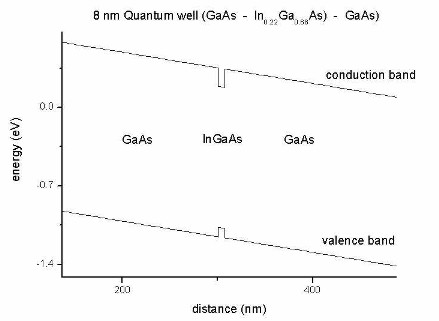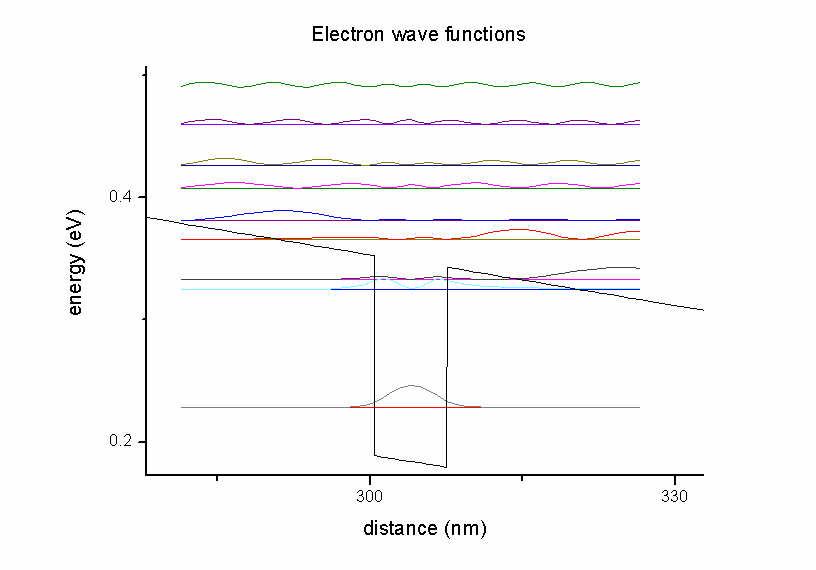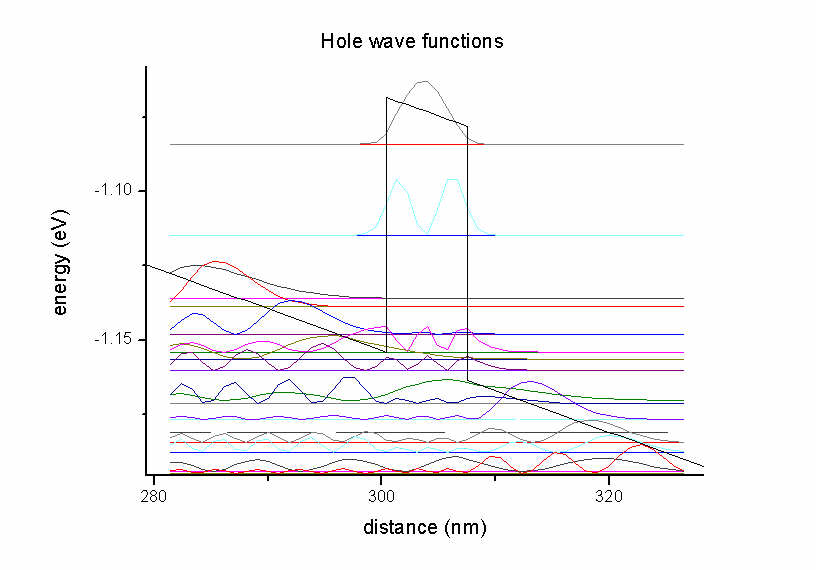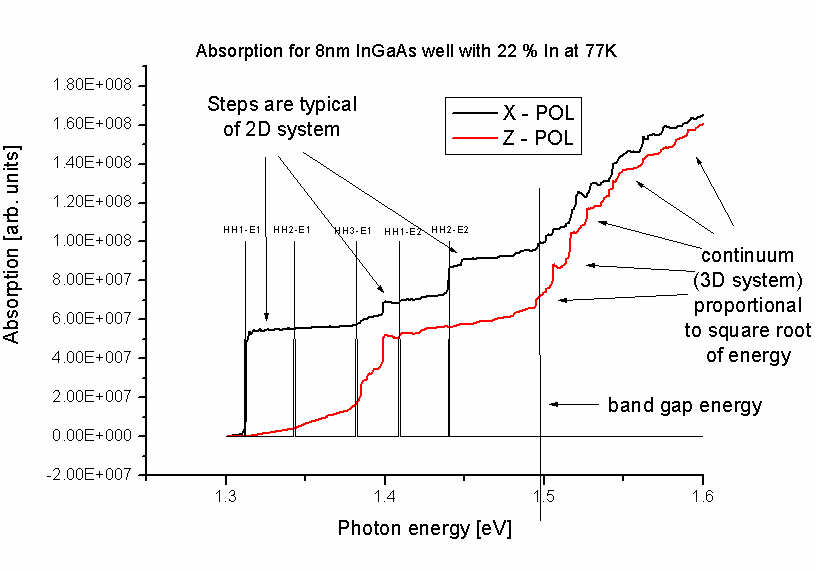The units of the optical absorption are [1/m] and not "arbitrary units" as indicated in the figure.

• The electric susceptibility tensor is contained in the file``` susceptibility_tensor.dat```:
```chi11re  chi11im  chi22re  chi22im  chi33re  chi33im  chi12re  chi12im  chi13re  chi13im  chi23re  chi23im ```Note:  As this tensor is complex, for each component, two values are written out.
`     re`: real part, `im`: imaginary part
`     `The relevant part for the absorption is only the imaginary part.

• In step 3, for each electron (`el`) and for each hole (`hl`) eigenvalue (i.e.` ev00*`), the energy dispersion E(kx,ky) is written out to the following files:
```optics/kp_optics_el_dispersionAVS2D_ev001.fld, *.coord, *.dat ... optics/kp_optics_hl_dispersionAVS2D_ev001.fld, *.coord, *.dat ... ```
The format of the files is compatible to the AVS/Express software.

The following figure shows the energy dispersion E(kx,ky) of the highest hole eigenstate (ground state).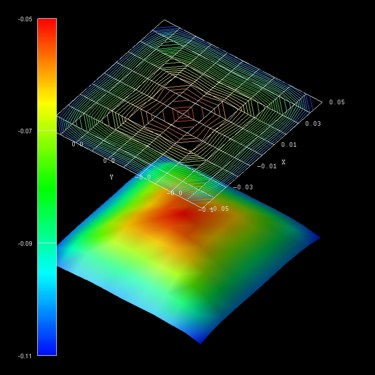The units of the k|| space grid coordinates kx and ky are [1/Angstrom] and the energy units are [eV].

The files
```- el_dispersion_100.dat - el_dispersion_110.dat - hl_dispersion_100.dat - hl_dispersion_110.dat```
show the same data but with slices along the  (i.e.  k||=(kx,ky=0)) and  (i.e. kx=ky) directions in k|| space.
Here all electron and all hole eigenvalues are contained in one file, respectively.

### Restrictions

• Only Dirichlet boundary conditions are supported so far.
• Step 2 and step 3 only work if``` raw-potential-in = yes```.

## Solar cells

The following flags are useful for solar cell simulations (photovoltaics).

``` incident-light-along-direction =  x          ! incident light along  x direction                                 =  y          ! incident light along  y direction                                 =  z          ! incident light along  z direction                                 = -x          ! incident light along -x direction                                 = -y          ! incident light along -y direction                                 = -z          ! incident light along -z direction ```In a 1D simulation, this specifier is optional. For 2D, and 3D, a direction must be specified.

### Solar spectrum

For a solar cell simulation, one has to read in a solar spectrum, e.g. AM 1.5, or AM 1.0 (AM = air mass).
They can be obtained from NREL website, e.g. ASTM-E490: https://www.nrel.gov/grid/solar-resource/spectra-astm-e490.html
(AMST = American Society for Testing and Materials)

```  import-solar-spectrum           = yes                                  = no     !file-solar-spectrum             = H:\solar_cells\ASTMG173_AM10.dat  ! ```AM 1.0 spectrum (extraterrestrial)``` !file-solar-spectrum             = H:\solar_cells\ASTMG173_AM15.dat  ! ```AM 1.5 spectrum```  file-solar-spectrum             = H:\solar_cells\ASTMG173_AM15G.dat ! ```AM 1.5G spectrum```  ```The file must consist of two columns (wavelength and spectrum), the units are` [nm] `and` [W/m^2*nm^-1]`.```    wavelength[nm]    AM1.5[W/m^2*nm^-1]    ...               ...```

```  number-of-suns                  =    1.0                 !    1   sun                                  =    2.5                 !    2.5 suns                                  =   10.0                 !   10   suns                                  = 1000.0                 ! 1000   suns ```To increase the power of the solar spectrum in order to model concentrator solar cells.

### Absorption coefficient

``` import-absorption-spectrum      = yes                                  = no      file-absorption-spectrum        = AbsorptionCoefficient_GaAs_300K.dat  ```The file must consist of two columns (wavelength and absorption coefficient), the units are` [nm] `and` [1/cm]`.```    wavelength[nm]    absorption[1/cm]    ...               ...```

### Reflection coefficient

Fraction of incident photons that are reflected from surface for a particular wavelength.

``` import-reflectivity-spectrum    = yes                                  = no      file-reflectivity-spectrum      = ReflectionCoefficient_GaAs_300K.dat  ```The file must consist of two columns (wavelength and reflection coefficient), the units are` [nm] `and` []`.```    wavelength[nm]    reflectivity[]    ...               ...```

### Transmission coefficient

Fraction of incident photons that are transmitted through the device for a particular wavelength (relevant for very thin devices).

```  import-transmission-spectrum    = yes                                  = no      file-transmission-spectrum      = ReflectionCoefficient_GaAs_300K.dat  ```The file must consist of two columns (wavelength and transmission coefficient), the units are` [nm] `and` []`.```    wavelength[nm]    transmission[]    ...               ...```

### Black body spectrum

```  calculate-black-body-spectrum = yes                                   = no     ```Calculates black body spectrum according to Planck's law, e.g. to compare the solar spectrum to the spectrum of a black body at T = 5778 K.

- The spectral energy density
- the spectral radiance (which is emitted per m2 and per unit solid angle sr (sr = steradian)) and
is calculated.

spectral energy density = spectral radiance * 4pi/c

There are several output files, i.e. output with respect to

• wavelength lambda in units of [m].

``` BlackBody_SpectralEnergyDensity_wavelength.dat   Wavelength[nm]                      SpectralEnergyDensity[kJ/m^3/m]```
``` BlackBody_SpectralRadiance_wavelength.dat   Wavelength[nm]                      SpectralRadiance[kW/m^2/nm/sr]``` ``` BlackBody_SpectralIrradiance_wavelength.dat   Wavelength[nm]                      SpectralIrradiance[kW/m^2/nm]```

• angular frequency w = 2pi v in units of [1/s].

``` BlackBody_SpectralEnergyDensity_angular_frequency.dat   AngularFrequency_omega[10^15/s]     SpectralEnergyDensity[10^-15J/m^3/s^-1]```
``` BlackBody_SpectralRadiance_angular_frequency.dat   AngularFrequency_omega[10^15/s]     SpectralRadiance[10^-12W/m^2/s^-1/sr]```
```  AngularFrequency_omega[10^15/s]     SpectralIrradiance[10^-12W/m^2/s^-1]```

• frequency v in units of [Hz].

``` BlackBody_SpectralEnergyDensity_frequency.dat   Frequency[THz]                      SpectralEnergyDensity[10^-15J/m^3/Hz]```
``` BlackBody_SpectralRadiance_frequency.dat   Frequency[THz]                      SpectralRadiance[10^-12W/m^2/sr]```
``` BlackBody_SpectralIrradiance_frequency.dat   Frequency[THz]                      SpectralIrradiance[10^-12W/m^2/Hz]```

• photon energy E = h v in units of [eV].

``` BlackBody_SpectralEnergyDensity_energy.dat   AngularFrequency_omega[10^15/s]     SpectralEnergyDensity[kJ/m^3/eV]```
``` BlackBody_SpectralRadiance_energy.dat   AngularFrequency_omega[10^15/s]     SpectralRadiance[kW/m^2/eV/sr]```
``` BlackBody_SpectralIrradiance_energy.dat   AngularFrequency_omega[10^15/s]     SpectralIrradiance[kW/m^2/eV]  ```

The file` BlackBody_Info.txt `contains some additional information about the calculated black body spectrum.

### Solar cell output

All output is twofold:
- one is with respect to wavelength in units of [nm]
- one is with respect to photon energy in units of [eV]  (indicated by``` _eV*.dat```)

• ```optics/Absorption_coefficient.dat               ```(as read in from file but now in units of [1/m])``` optics/Absorption_coefficient_interpolated.dat  ```(interpolated on wavelength grid of solar spectrum but now in units of [1/m])``` optics/Reflectivity.dat                         ```(as read in from file)``` optics/Reflectivity_interpolated.dat            ```(interpolated on wavelength grid of solar spectrum)``` optics/Transmission.dat                         ```(as read in from file)``` optics/Transmission_interpolated.dat            ```(interpolated on wavelength grid of solar spectrum)
``` optics/SolarSpectralIrradiance_sun0001.dat      ```(as read in from file)``` optics/PhotonFlux_sun0001.dat                   ```(photon flux density calculated from solar spectrum)``` ```Total number of photons in the solar spectrum above an energy value contributing to the maximum photo current for a solar cell made with a specific band gap:``` optics/PhotonFlux_BandGap_eV_sun0001.dat        ```(calculated from solar spectrum)``` optics/PhotoCurrent_BandGap_eV_sun0001.dat      ```(calculated from solar spectrum)``` optics/SpectralResponse_sun0001.dat             external and internal spectral response optics/QuantumEfficiency_sun0001.dat            external and internal quantum efficiency optics/GenerationRateLight_AVS_sun0001.fld      2D plots GenerationRate(x,lambda) and  GenerationRate(x,E) optics/GenerationRateLight_sun0001.dat          1D plot  GenerationRate(x) optics/GenerationRate_eV_sun0001.dat            1D plot  GenerationRate(E)  (E=energy) optics/GenerationRate_Wavelength_sun0001.dat    1D plot  GenerationRate(lambda)  ```
• ```current/IV_characteristics_new.dat voltage[V]  current[A/m^2] ... power[W/m^2] powersolar[W/m^2] efficiency[%]  ```
• ```  ****************************************************************************************  Solar cell results  ****************************************************************************************  short-circuit current:       I_sc    = 281.473346 [A/m^2]                  (photo current: It increases with smaller band gap.)  open-circuit voltage:        U_oc    =  -1.012500 [V]                      (U_oc <= built-in potential ~ band gap)  current at maximum power:    I_max   = 273.089897 [A/m^2]  voltage at maximum power:    U_max   =  -0.925000 [V]  maximum power output:        P_max   = U_max * I_max = -252.608155 [W/m^2] (condition for maximum power output: dP/dV = 0)  maximum extracted power:     P_solar = - P_max       =  252.608155 [W/m^2]  incident power:              P_in    =   0.000000 [W/m^2]  ideal conversion efficiency: eta     = P_max / P_in  = Infinity %  fill factor:                 FF      =   0.886370  In practice, a good fill factor is around 0.8.  All these results are approximations.  They are only correct if a lot of voltage steps have been used (i.e. a high resolution).  ****************************************************************************************  ```